# ISEE Middle Level Math : Outcomes

## Example Questions

← Previous 1 3 4 5 6 7 8

### Example Question #1 : How To Find The Probability Of An Outcome

There are 32 marbles in a bag: 7 are Red, 11 are Blue, 8 are Purple and 6 are Green. If I pick one marble out of the bag, which color would it most likely be?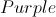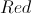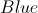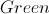Explanation:

Find the color with the largest number of marbles in the bag.

Answer: There are more blue marbles in the bag, therefore it would be more likely to pick a blue marble.

### Example Question #1 : Outcomes

The red queens are removed from a standard deck of fifty-two cards. What is the probability that a card randomly drawn from that modified deck will be a face card (jack, queen, king)?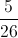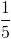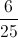Explanation:

There are four cards of each rank in a standard deck; since three ranks - jacks, queens, kings - are considered face cards, this makes twelve face cards out of the fifty-two. But two of those face cards - two red queens - have been removed, so now there are ten face cards out of fifty. This makes the probability of a randomly drawn card being a face card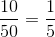.

### Example Question #772 : Grade 7

Jamie rolled a normal 6-sided die. What is the probability of rolling a number greater than 4?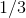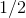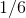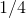Explanation:

Probability is determined by dividing the number of incidences of a specific outcome (in this case rolling greater than 4, or rolling a 5 or 6) by the total number of outcomes (there are 6 sides to the die).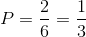### Example Question #2 : Develop And Compare Probability Models And Find Probabilities Of Events: Ccss.Math.Content.7.Sp.C.7

What is the probability of drawing a diamond from a regular set of playing cards?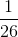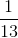Explanation:

Probability is determined by dividing the number of outcomes of a particular event by the total number of events. In a regular deck of playing cards, there are 52 cards and 13 diamonds.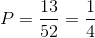### Example Question #1 : Probability

What is the probability of drawing a red jack from a standard deck of playing cards?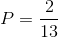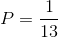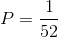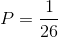Explanation:

A standard deck containscards includingjacks,of which are red. Therefore, the probability is: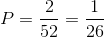### Example Question #773 : Grade 7

There are 5 yellow pebbles, 12 orange pebbles, and 8 red pebbles.  What is the probability of picking a red or yellow pebble?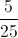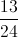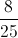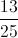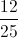Explanation:

There are 13 yellow and red pebbles.  There are 25 pebbles total.  Therefor the probability of choosing a red or yellow pebble is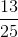.

### Example Question #1 : Probability

There are 6 red marbles, 4 purple marbles, and 9 green marbles in a bag.  What is the probablility of randomly drawing a green marble?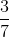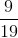Explanation:

First we need to determine the total number of marbles in the bag: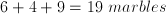There are 9 green marbles, so the probability of drawing one of those 9 marbles out of 19 possible marbles is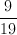.

### Example Question #2 : Probability

A bag containsred marbles,pink marbles, andpurple marbles. What is the probability of not choosing a purple marble?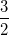Explanation:

The probability (p) is equal to the number of a specific event (purple marbles) divided by the total number of events. The probability of NOT p (called q) is equal to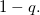In this example: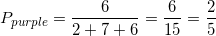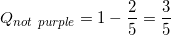### Example Question #1 : Probability

A bag of marbles contains 8 black marbles, 4 red marbles, 3 blue marbles, and 5 white marbles. What is the probability of not drawing a black marble?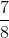Explanation:

Probability is determined by dividing the possible number of instances of a particular outcome by the total number of possible outcomes. Therefore, we need to find the probability of drawing red, blue, or white divided by the total number of outcomes.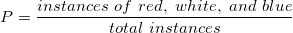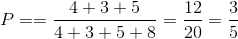### Example Question #821 : Isee Middle Level (Grades 7 8) Mathematics Achievement

If you meet a random person on the street, what is the probability he or she was born on a weekend?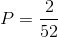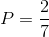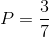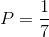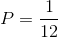Explanation:

There are seven days in a week.

The probability that a person you meet was born on any particular day is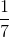.

The question asks for the probability that the person you meet was born on a weekend (Saturday or Sunday). Therefore, we must add the probability of being born on either day: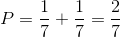← Previous 1 3 4 5 6 7 8

### All ISEE Middle Level Math Resources